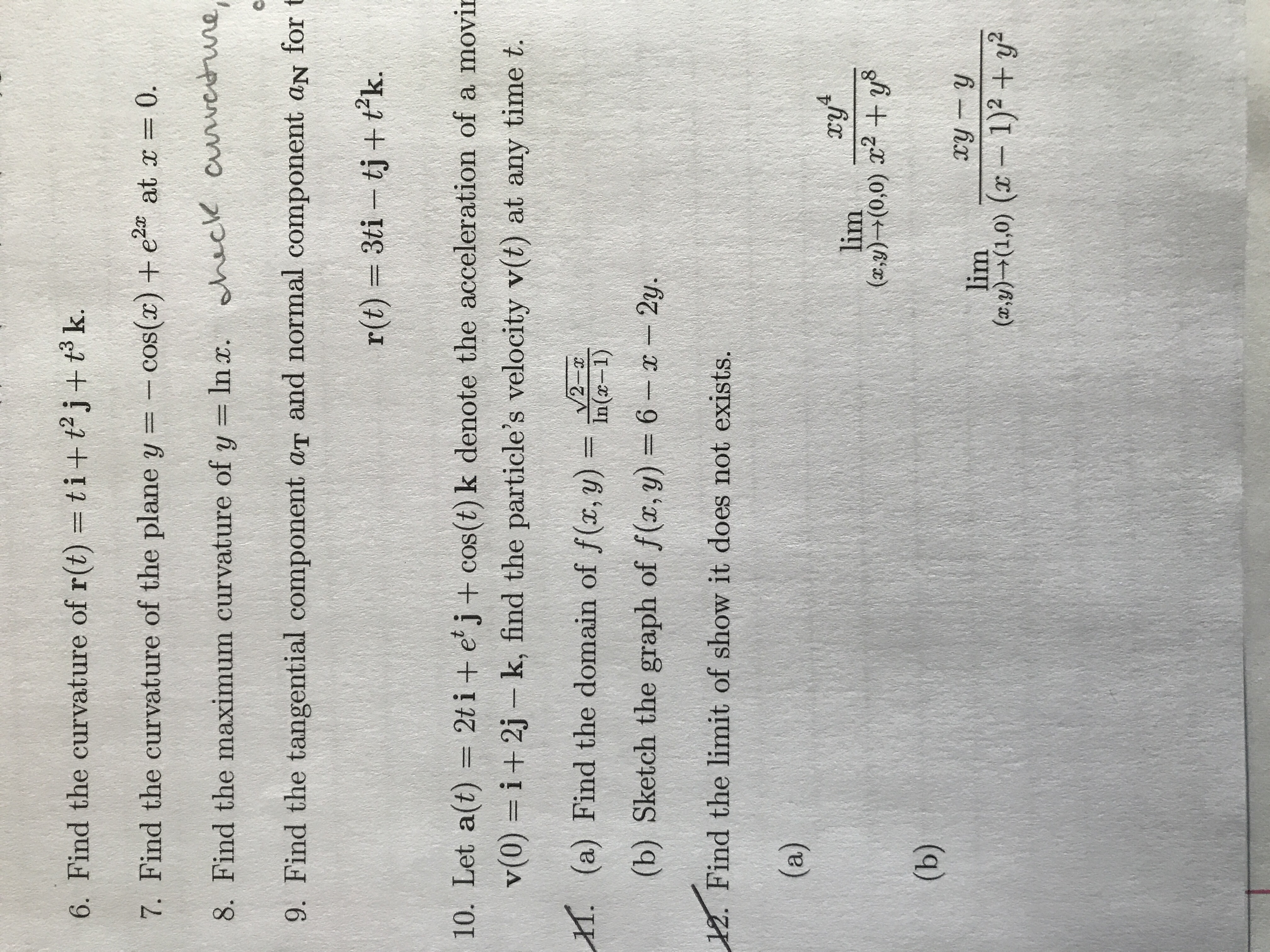# 6. Find the curvature of r(t) = ti + t2j +t k.at r = 0.7. Find the curvature of the plane y = -cos() + e8. Find the maximum curvature of y In . nack cuwerune,9. Find the tangential component aT and normal component aN for3ti tj + tk.r(t)10. Let a(t) = 2t i + e j + cos (t) k denote the acceleration of a movirv(0) i+2j- k, find the particle's velocity v(t) at any time t.. (a) Find the domain of f(x, y) =nV2 x(b) Sketch the graph of f(r, y) = 62y.12. Find the limit of show it does not exists.(a)lim(xy)+(0,0) +y8(b)ry ylim(a)(1,0) (1)2+y2

Question
28 views

Help w/ #6help_outlineImage Transcriptionclose6. Find the curvature of r(t) = ti + t2j +t k. at r = 0. 7. Find the curvature of the plane y = -cos() + e 8. Find the maximum curvature of y In . nack cuwerune, 9. Find the tangential component aT and normal component aN for 3ti tj + tk. r(t) 10. Let a(t) = 2t i + e j + cos (t) k denote the acceleration of a movir v(0) i+2j- k, find the particle's velocity v(t) at any time t. . (a) Find the domain of f(x, y) =n V2 x (b) Sketch the graph of f(r, y) = 6 2y. 12. Find the limit of show it does not exists. (a) lim (xy)+(0,0) +y8 (b) ry y lim (a)(1,0) (1)2+y2 fullscreen
check_circle

Step 1

The curve is given by ti+t2j+t3k.

The formula to calculate the curvature of the curve is given by,

Step 2

The r’(t) is given by differentiating r(t) with respect to t.

then,

Step 3

T(t) is give...

### Want to see the full answer?

See Solution

#### Want to see this answer and more?

Solutions are written by subject experts who are available 24/7. Questions are typically answered within 1 hour.*

See Solution
*Response times may vary by subject and question.
Tagged in

### Integration